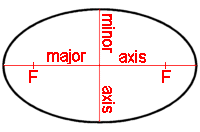Cleave Books
The Ellipses Calculator
 Show values to . . . 3 4 5 6 7 8 9 significant figures.
 major axis = units minor axis = unitseccentricity = area = square units foci distance = units loop length = units *perimeter* = units
Remember: Appropriate units need to be attached.
*perimeter* value is only approximate. (see Notes)
Very large and very small numbers appear in e-Format.
Unvalued zeros on all numbers have been suppressed.
The original inputs have NOT been adjusted in any way.
A note on Format and Accuracy is available.

 Additional InformationThe ellipse has two principal lines which are at right angles to, and bisect, each other. One determines its maximum 'length' and is known as the major axis. The other fixes its minimum 'width' and is known as the minor axis. Each axis is also a line of symmetry. These axes might be thought of as corresponding to two diameters of a circle, but in a circle ALL the diameters are the same length.The 'centre' of an ellipse is the point where the two axes cross. But, more important are the two points which lie on the major axis, and at equal distances from the centre, known as the foci (pronounced 'foe-sigh'). The distance between these two points is given in the calculator as the foci distance. In the diagram, the two foci (for that particular ellipse) are marked F.The eccentricity of an ellipse is a measure of how fat (or thin) it is. Its value can vary from 0 to 1. A value of 0 (major and minor are equal in length) indicates it is a circle. A value of 1 means the minor axis does not exist, so the ellipse collapses into a straight line.An ellipse is often described as a 'squashed' or 'stretched' circle, in fact it belongs to a family of curves known as the 'conic sections' (the circle is also one of that family) and the circle can be considered as a special case of the ellipse.Remember that once the size of an ellipse has been fixed then its exact shape is mathematically determined. In other words, the line forming the perimeter can be drawn in only ONE way. This is distinct from an oval where the perimeter has only to be a concave curve, and there are many possibilities. Simply, an ellipse IS an oval, but an oval may or may not be an ellipse. PerimeterUnlike a circle, the length of the perimeter of an ellipse cannot be found easily. (In fact it was that problem which gave rise to the invention of 'elliptic integrals'.) However, there are several approximation formulas and the one used here (first published by Ramanujan in 1914) gives a very close solution. Note that it cannot be entered as one of the pieces of data.Drawing an ellipse by the 'Loop method'There are many ways of drawing an ellipse but one of the simplest is to mark the two foci on a piece of paper, put a pin in each, drop a circular loop of string over these pins, put the point of a pencil inside the loop, then move the pencil over the paper, keeping the string taut all the time. Easier to do than to describe! The calculator gives the size of the loop (against loop length) required to draw any particular ellipse. CautionsIf a value of 0 (zero) is given, it means that a solution is possible but is too small to be of any real use.A statement that 'No solution was found' means exactly that. If a solution is possible, then it must lay outside the limits set within the calculator.

Go to Cleave Books main Index